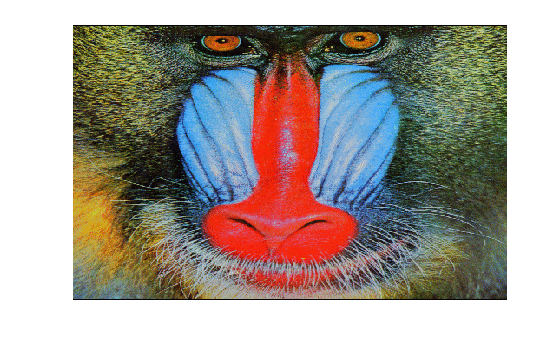Documentation

# im2frame

Convert image to movie frame

## Syntax

``F = im2frame(RGB)``
``F = im2frame(X,map)``
``F = im2frame(X)``

## Description

example

````F = im2frame(RGB)` converts the truecolor image, `RGB`, into a movie frame `F`.```
````F = im2frame(X,map)` converts the indexed image, `X`, and the associated colormap, `map`, into a movie frame `F`.```
````F = im2frame(X)` converts the indexed image, `X`, into a movie frame `F` using the current colormap.```

## Examples

collapse all

Load an indexed image of a mandrill's face.

`load mandrill`

Display the image `X` using its associated colormap, `map`, which has 220 colors.

```figure image(X) colormap(map) axis off```Make a movie that shows the effect of reducing the number of colors of the image. There will be eight movie frames in total. Preallocate an array of structures to store the movie frames.

`F(8) = struct('cdata',[],'colormap',[]);`

In a loop, reduce the number of colors in the indexed image by using the `imapprox` function. Use the `im2frame` function to convert the images into frames of a movie. The first frame is the original image with all 220 colors. The second frame has 128 colors. Each successive frame has half the number of colors. The last frame has the minimum number of colors, 2.

```for j = 1:8 q = 2^(9-j); [Y,newmap] = imapprox(X,map,q,'nodither'); F(j) = im2frame(Y,newmap); end```

To play the movie twice with a frame rate of three frames per second, use `movie(F,2,3)`.

## Input Arguments

collapse all

Truecolor image, specified as an m-by-n-by-3 numeric array. If you specify an image of data type `double`, then values must be in the range [0, 1].

Data Types: `double` | `uint8`

Indexed image, specified as an m-by-n matrix of integers.

Data Types: `double` | `uint8`

Colormap associated with indexed image `X`, specified as a c-by-3 numeric matrix with values in the range [0, 1]. Each row of `map` is a three-element RGB triplet that specifies the red, green, and blue components of a single color of the colormap.

Data Types: `double`

## Output Arguments

collapse all

Movie frame, returned as a structure with two fields:

• `cdata` — The image data stored as an array of `uint8` values.

• `colormap` — The colormap. For truecolor (RGB) images, this field is empty.